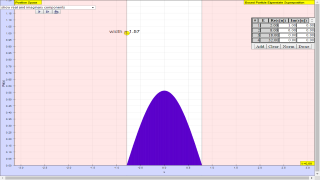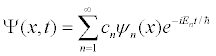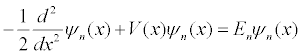### Translations

Code Language Translator Run### Software Requirements

SoftwareRequirements

 Android iOS Windows MacOS with best with Chrome Chrome Chrome Chrome support full-screen? Yes. Chrome/Opera No. Firefox/ Samsung Internet Not yet Yes Yes cannot work on some mobile browser that don't understand JavaScript such as..... cannot work on Internet Explorer 9 and below

### CreditsWolfgang Christian

### end faq

http://iwant2study.org/lookangejss/06QuantumPhysics/ejss_model_BoundEigenstateSuperpositionwee/BoundEigenstateSuperpositionwee_Simulation.xhtml

[text]

# The Superposition Principle

The fundamental building blocks of one-dimensional quantum mechanics are energy eigenfunctions ψn(x) and energy eigenvalues En. For a given potential energy function V(x) and boundary conditions, energy eigenfunctions can be determined either analytically or numerically. Most of the time in quantum mechanics these energy eigenfunctions are determined in position space. Once these energy eigenstates are determined, more interesting quantum-mechanical wave functions Ψ(x,t) can be studied by applying the superposition principlewhere the expansion coefficients cn satisfy Σ cn|2 = 1. Depending on how many of the coefficients cn are non-zero, one may have an energy eigenstate, a two-state superposition, or even an initially localized (usually Gaussian shaped) wave packet.

Any complete orthonormal set of eigenfunctions can be used to construct the wave function Ψ(x,t). This simulation uses the superposition principle to construct and display a time-dependent wave function using either infinite square well (ISW) or simple harmonic oscillator (SHO) eigenfunctions.

## Units

Although the metric (MKS) system of units has become the standard international system of units, it is not well suited for computation if the quantities being computed are very large or very small. Quantum phenomena occurs on the microscopic scale at very fast times and computations are usually done using an atomic system of units in which the reduced Plank's constant ħ, the Bohr radius ao, and the mass of the electron m are set equal to unity. The one-dimensional time independent Schrödinger equation in these units is:In atomic units, one unit time is 2.42×10-17 seconds, one unit of distance is 5.29×10-11 meters, and one unit of energy is 4.36×10-18 Joules. This simulation models a particle with the mass of an electron using these atomic units.

### References:

• For an excellent tutorial on energy eigenfunction shape and the relationship to the potential energy function, see: A. P. French and E. F. Taylor, Qualitative plots of bound state wave functions, Am. J. Phys. 39, 961-962 (1971).
• An Introduction to Quantum Mechanics (2ed) by David J. Griffiths page 28.

### Credits:

The Bound Energy Eigenstate Superposition JavaScript simulation was developed by Wolfgang Chrsitian using the Easy Java/JavaScript Simulations (EjsS) modeling tool. You can examine and modify this simulation if you have EjsS version 5.2 or above installed by importing the model's zip archive into EjsS. Information about EjsS is available at: <http://www.um.es/fem/Ejs/> and in the OSP ComPADRE collection <http://www.compadre.org/OSP/>.

[text]

[text]

### Version:

1. http://weelookang.blogspot.com/2016/06/bound-eigenstate-superposition-by.html
2. http://www.compadre.org/osp/items/detail.cfm?ID=13742 original simulation by Wolfgang Christian

[text]

### end faq

Rating 0.00 (0 Votes)
Parent Category: Physics
Category: 06 Quantum Physics
Hits: 3768